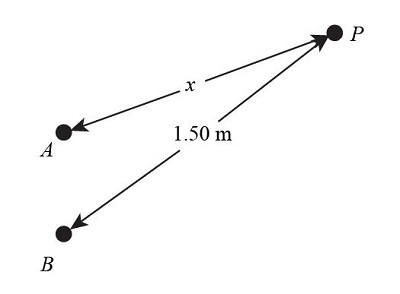# Two small stereo speakers A and B that are 1.4 m apart are sending out sound of wavelength 34 cm...

## Question:

Two small stereo speakers A and B that are 1.4 m apart are sending out sound of wavelength 34 cm in all directions and all in phase. A person at point P starts out equidistant from both speakers and walks so that he is always 1.5 m from speaker B. For what values x will the sound this person hears be

(a) Maximally reinforced,

(b) Limit your solution to the cases where x is less then or equal to 1.5 m.

## Wavelength:

The wavelength can be expressed as the range across two continuous crests. Mathematically, the wavelength can be evaluated as the inverse of the frequency. It means with an increase in frequency, the value of the wavelength of wave decreases.

Given data:

• Wavelength, {eq}\lambda = 34\;{\rm{cm}} = 0.34\;{\rm{m}} {/eq}
• Distance between person and speaker B, {eq}{r_2} = 1.5\;{\rm{m}} {/eq}

The person is at distance {eq}x {/eq} from A and at a distance of {eq}1.5\;{\rm{m}} {/eq} from B.

{eq}{r_2} - {r_1} = 1.5 - x {/eq}

Here, {eq}{r_1} = x {/eq}Part a)

The condition of maximum reinforcement is:

{eq}\begin{align*} {r_2} - {r_1} &= m\lambda \\ 1.5 - x &= m \times 0.34\\ x &= 1.5 - 0.34m......................(1) \end{align*} {/eq}

The maximum reinforcement occurs when, {eq}m = 0 {/eq}

So,

{eq}\begin{align*} x &= 1.5 - 0.34m\\ x &= 1.5\;{\rm{m}} \end{align*} {/eq}

Therefore maximum reinforcement occurs at {eq}x = 1.5\;{\rm{m}} {/eq} .

Part b)

When, {eq}m = 1 {/eq}

Substitute this value in equation (1)

{eq}\begin{align*} x &= 1.5 - 0.34m\\ x &= 1.5 - 0.34\\ x &= 1.16\;{\rm{m}} \end{align*} {/eq}

When, {eq}m = 2 {/eq}

{eq}\begin{align*} x &= 1.5 - 0.34m\\ x &= 0.82\;{\rm{m}} \end{align*} {/eq}

When, {eq}m = 3 {/eq}

{eq}\begin{align*} x &= 1.5 - 0.34m\\ x &= 0.48\;{\rm{m}} \end{align*} {/eq}

When, {eq}m = 4 {/eq}

{eq}\begin{align*} x &= 1.5 - 0.34m\\ x &= 0.14\;{\rm{m}} \end{align*} {/eq}

Therefore, the values of {eq}x {/eq} are {eq}1.16\;{\rm{m,}}\;{\rm{0}}{\rm{.82}}\;{\rm{m,}}\;{\rm{0}}{\rm{.48}}\;{\rm{m,}}\;{\rm{0}}{\rm{.14}}\;{\rm{m}} {/eq} .